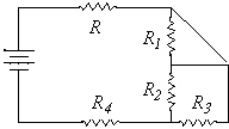# College Physics Study Set 7

Physics & Astronomy

## Quiz 18 : Direct-Current CircuitsStudy FlashcardsLooking for Introductory Physics Homework Help?

## Quiz 18 :Direct-Current Circuits

Question TypeThree resistors, each with resistance R1, are in series in a circuit.They are replaced by one equivalent resistor, R.Comparing this resistor to the first resistor of the initial circuit, which of the following is true?
Free
Multiple Choice

A

Tags
Choose question tagThree 8.0-W resistors are connected in parallel.What is their equivalent resistance?
Free
Multiple Choice

D

Tags
Choose question tagThe basic function of an electromotive force in a circuit is to do which of the following?
Free
Multiple Choice

B

Tags
Choose question tagThree resistors, with values of 2.0, 4.0 and 8.0 W, respectively, are connected in series.What is the overall resistance of this combination?
Multiple Choice
Tags
Choose question tagWhen a 24.0-W resistor is connected across a 12.0-V battery, a current of 482 mA flows.What is the power output delivered by the emf of the battery?
Multiple Choice
Tags
Choose question tagWhen a light bulb is turned on, its resistance increases until it reaches operating temperature. What happens to the current in the bulb as it is warming up?
Multiple Choice
Tags
Choose question tagWhen a 24.0-W resistor is connected across a 12.0-V battery, a current of 482 mA flows.What is the internal resistance of the battery?
Multiple Choice
Tags
Choose question tagThree resistors connected in series each carry currents labeled I1, I2 and I3.Which of the following expresses the value of the total current IT in the system made up of the three resistors in series?
Multiple Choice
Tags
Choose question tagIf R1 < R2 < R3, and if these resistors are connected in series in a circuit, which one dissipates the greatest power?
Multiple Choice
Tags
Choose question tagThree resistors connected in series have individual voltages labeled DV1, DV2 and DV3, respectively.Which of the following expresses the value of the total voltage DVT taken over the three resistors together?
Multiple Choice
Tags
Choose question tagWhich voltage is not caused by a source of emf?
Multiple Choice
Tags
Choose question tagThree 8.0-W resistors are connected in series.What is their equivalent resistance?
Multiple Choice
Tags
Choose question tagThe internal resistances of an ideal voltmeter and an ideal ammeter are respectively (ideal meaning the behavior of the system is not changed when using the meter):
Multiple Choice
Tags
Choose question tagThree 4.0-W resistors are connected in parallel to a 12.0-V battery.What is the current in any one of the resistors?
Multiple Choice
Tags
Choose question tagThe two ends of a 3.0-W resistor are connected to a 9.0-V battery.What is the total power delivered by the battery to the circuit?
Multiple Choice
Tags
Choose question tagWhich resistor is in series with resistor R?Multiple Choice
Tags
Choose question tagResistors of values 8.0 W, 12.0 W, and 24.0 W are connected in series across a battery with a small internal resistance.Which resistor dissipates the greatest power?
Multiple Choice
Tags
Choose question tagWhen a 24.0-W resistor is connected across a 12.0-V battery, a current of 482 mA flows.What is the resulting terminal voltage of the battery?
Multiple Choice
Tags
Choose question tagThe two ends of a 3.0-W resistor are connected to a 9.0-V battery.What is the current through the resistor?
Multiple Choice